# Cross product

(diff) ← Older revision | Latest revision (diff) | Newer revision → (diff)

crossed product, of a groupand a ringAn associative ring defined as follows. Suppose one is given a mappingof a groupinto the isomorphism group of an associative ringwith an identity, and a family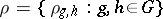of invertible elements of, satisfying the conditions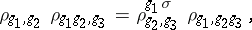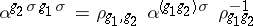for all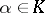and. The familyis called a factor system. Then the cross product ofandwith respect to the factor systemand the mappingis the set of all formal finite sums of the form(where the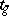are symbols uniquely assigned to every element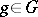), with binary operations defined by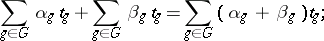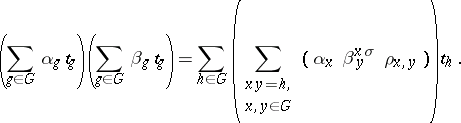This ring is denoted by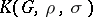; the elementsform a-basis of it.

Ifmapsonto the identity automorphism of, thenis called a twisted or crossed group ring, and if, in addition,for all, thenis the group ring ofover(see Group algebra).

Letbe a field anda monomorphism. Thenis a simple ring, being the cross product of the field with its Galois group.

How to Cite This Entry:
Cross product. Encyclopedia of Mathematics. URL: http://encyclopediaofmath.org/index.php?title=Cross_product&oldid=16831
This article was adapted from an original article by A.A. Bovdi (originator), which appeared in Encyclopedia of Mathematics - ISBN 1402006098. See original article